# Area of a Circle Circumference pi diameter Diameter

• Slides: 12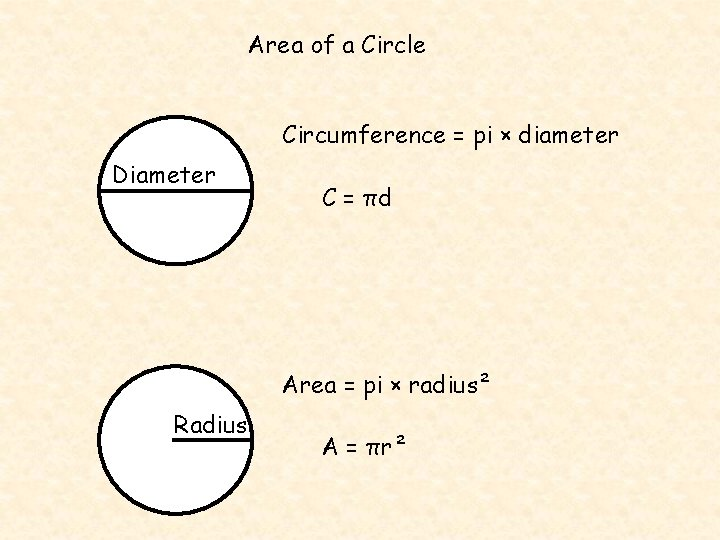Area of a Circle Circumference = pi × diameter Diameter C = πd Area = pi × radius² Radius A = πr²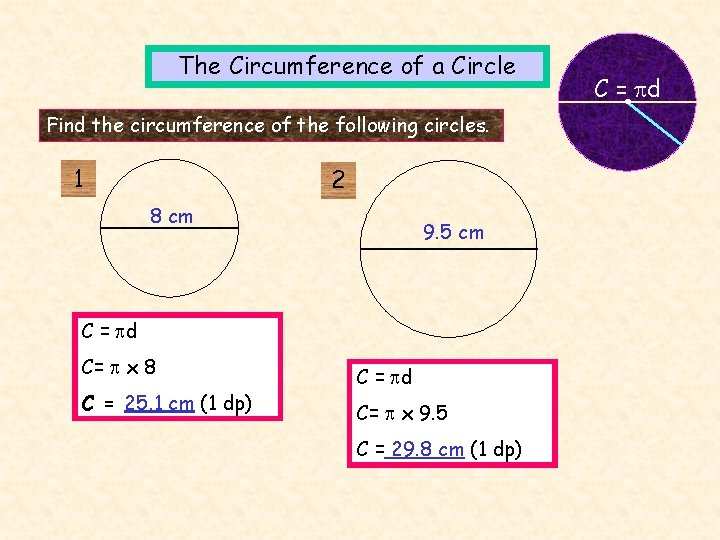The Circumference of a Circle Find the circumference of the following circles. 1 2 8 cm 9. 5 cm C = d C= x 8 C = 25. 1 cm (1 dp) C = d C= x 9. 5 C = 29. 8 cm (1 dp) C = dThe Circumference of a Circle C = d Find the circumference of the following circles. 4 3 3 mm 2. 1 m C = 2 r C=2 x x 3 C = 2 x x 2. 1 C = 18. 8 mm C = 13. 2 m (1 dp)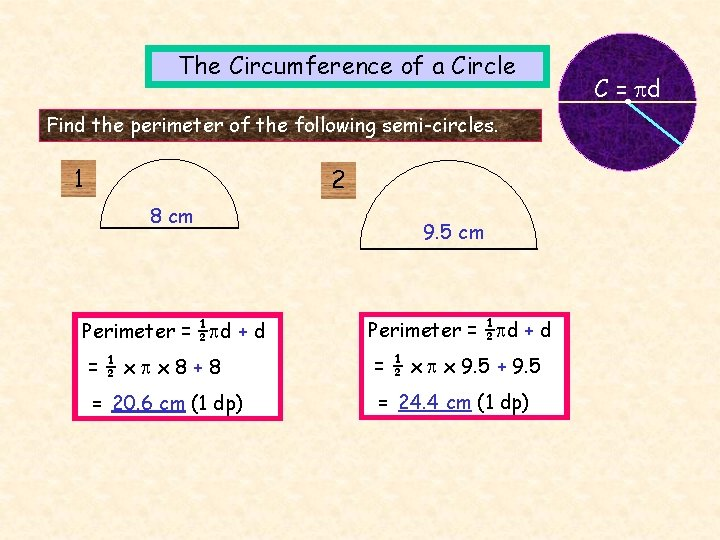The Circumference of a Circle Find the perimeter of the following semi-circles. 1 2 8 cm 9. 5 cm Perimeter = ½ d + d =½x x 8+8 = ½ x x 9. 5 + 9. 5 = 20. 6 cm (1 dp) = 24. 4 cm (1 dp) C = dThe Circumference of a Circle C = d Find the perimeter of the ¼ and ¾ circles. 3 4 6 cm Perimeter = ¼(2 r) + 2 r 8. 5 cm Perimeter = ¾(2 r) + 2 r =¼x 2 x x 6+2 x 6 = ¾ x 2 x x 8. 5 + 2 x 8. 5 = 21. 4 cm (1 dp) = 57. 1 cm (1 dp)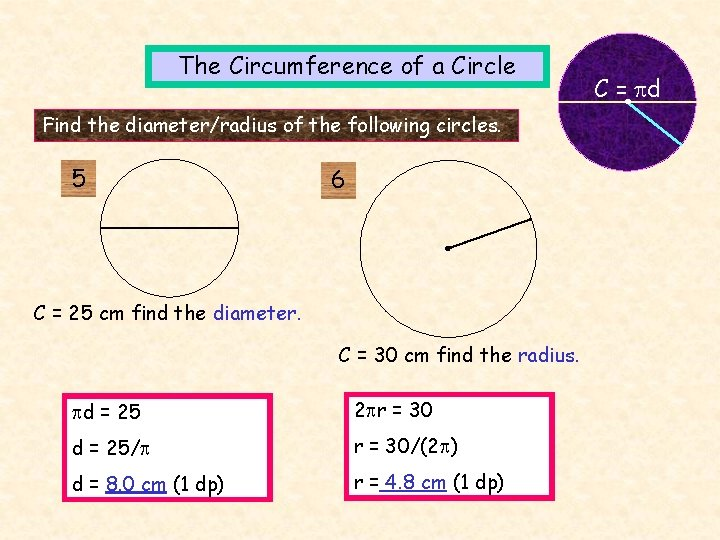The Circumference of a Circle Find the diameter/radius of the following circles. 5 6 C = 25 cm find the diameter. C = 30 cm find the radius. d = 25 2 r = 30 d = 25/ r = 30/(2 ) d = 8. 0 cm (1 dp) r = 4. 8 cm (1 dp) C = d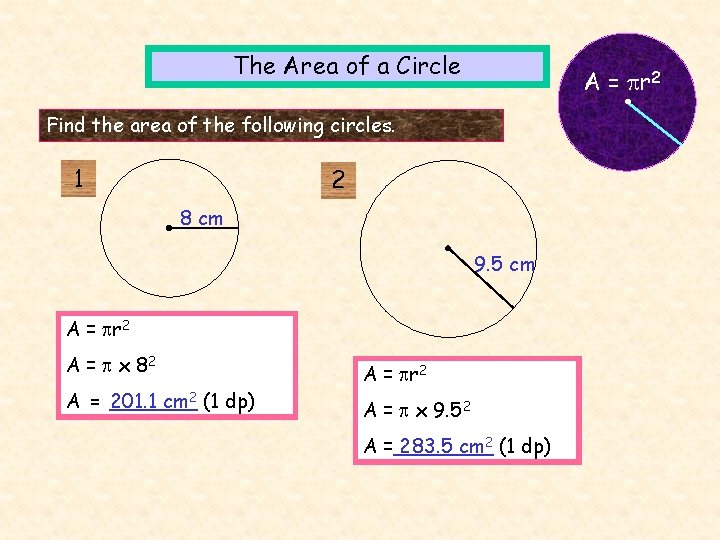The Area of a Circle A = r 2 Find the area of the following circles. 1 2 8 cm 9. 5 cm A = r 2 A = x 82 A = 201. 1 cm 2 (1 dp) A = r 2 A = x 9. 52 A = 283. 5 cm 2 (1 dp)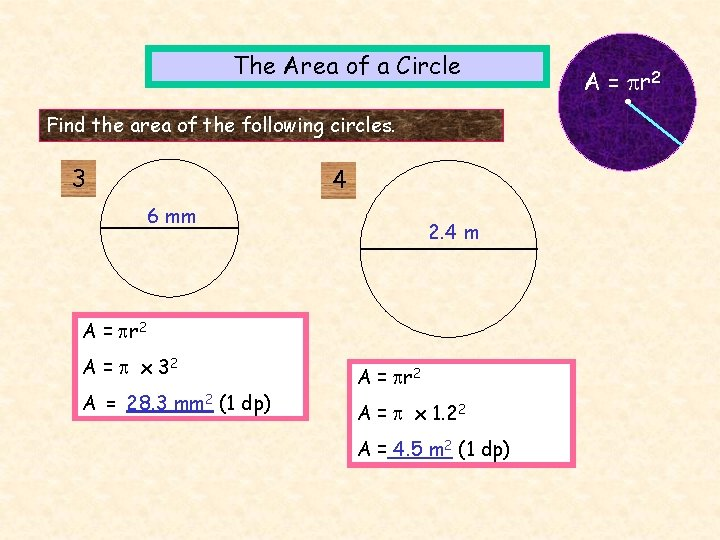The Area of a Circle Find the area of the following circles. 3 4 6 mm 2. 4 m A = r 2 A = x 32 A = 28. 3 mm 2 (1 dp) A = r 2 A = x 1. 22 A = 4. 5 m 2 (1 dp) A = r 2Find the area of the clock face and radar screen. A = r 2 12 cm A = r 2 A = x 122 A = 452. 4 cm 2 (1 dp) 60 cm A = r 2 A = x 302 A = 2827 cm 2 (nearest cm 2)The Area of a Circle Find the area of the following semi-circles. 1 2 8 cm 9. 5 cm A = ½ r 2 = ½ x x 42 = ½ x x 4. 752 = 25. 1 cm 2 (1 dp) = 35. 4 cm 2 (1 dp) A = r 2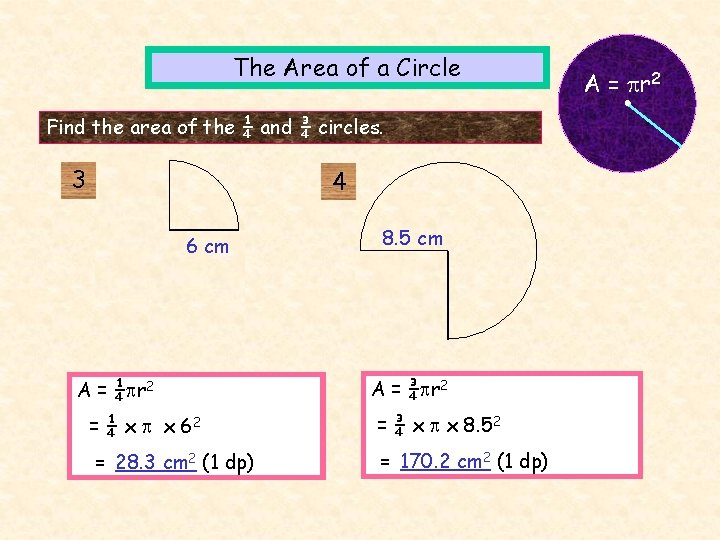The Area of a Circle Find the area of the ¼ and ¾ circles. 3 4 6 cm A = ¼ r 2 8. 5 cm A = ¾ r 2 = ¼ x x 62 = ¾ x x 8. 52 = 28. 3 cm 2 (1 dp) = 170. 2 cm 2 (1 dp) A = r 2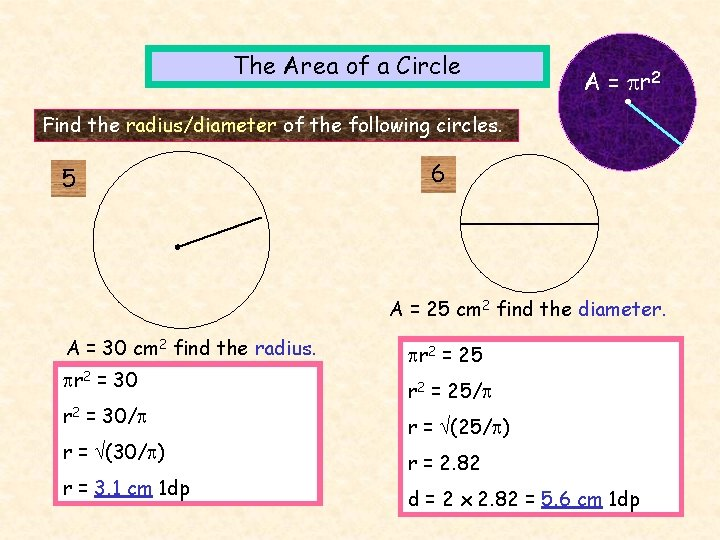The Area of a Circle A = r 2 Find the radius/diameter of the following circles. 5 6 A = 25 cm 2 find the diameter. A = 30 cm 2 find the radius. r 2 = 30/ r = (30/ ) r = 3. 1 cm 1 dp r 2 = 25/ r = (25/ ) r = 2. 82 d = 2 x 2. 82 = 5. 6 cm 1 dp